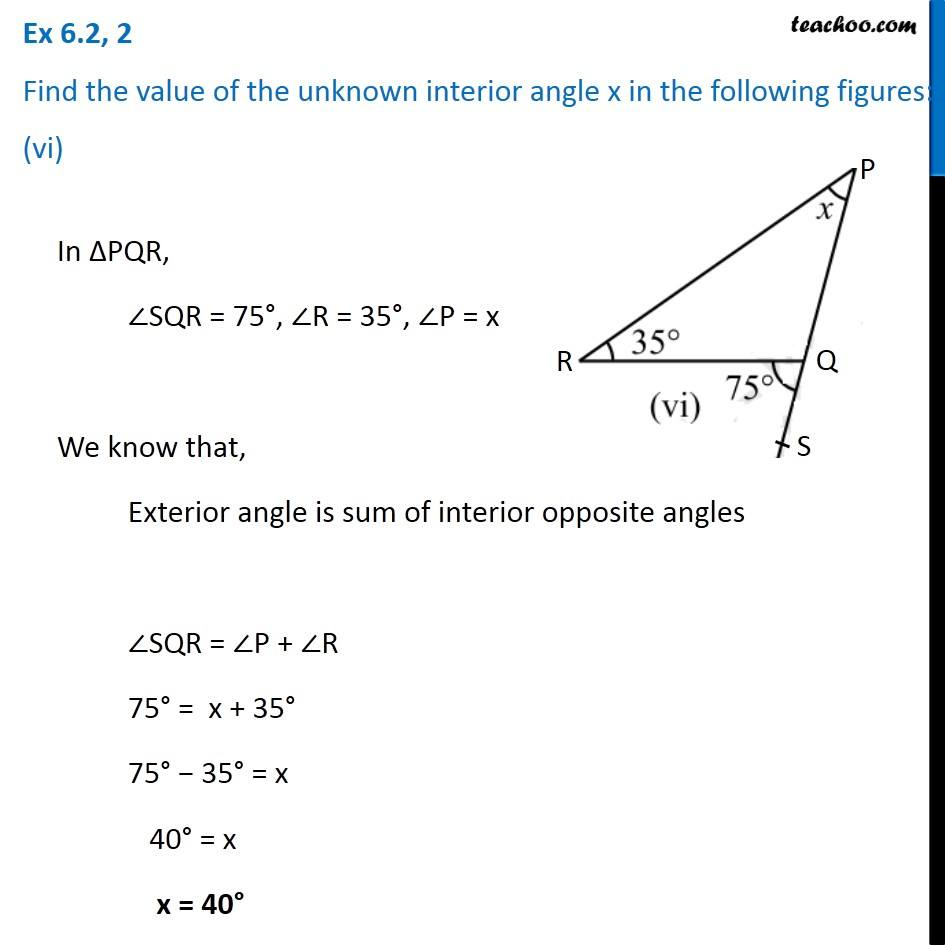Ex 6.2

Chapter 6 Class 7 Triangle and its Properties
Serial order wiseLearn in your speed, with individual attention - Teachoo Maths 1-on-1 Class

### Transcript

Ex 6.2, 2 Find the value of the unknown interior angle x in the following figures: (vi) In ∆PQR, ∠SQR = 75°, ∠R = 35°, ∠P = x We know that, Exterior angle is sum of interior opposite angles ∠SQR = ∠P + ∠R 75° = x + 35° 75° − 35° = x 40° = x x = 40°